Home/Blog/Cracking the top 40 Facebook coding interview questions

# Cracking the top 40 Facebook coding interview questions

Sep 24, 2020 - 24 min read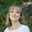Amanda FawcettLanding a job at Facebook is a dream for many developers around the globe. Facebook is one of the top tech companies in the world, with a workforce of over 52,000 strong. Facebook is known for its growth-based company culture, fast promotion tracks, excellent benefits, and top salaries that few companies can match.

But competition is fierce, and with a swell of new hires, Facebook is on the lookout for the top candidates. Facebook focuses on your cultural fit, generalist knowledge, ability to build within constraints, and expert coding skills.

To help you prepare, today I will walk through everything you need to crack an Facebook interview, including coding questions and a step-by-step preparation guide.

Today we will go over:

## Overview of the Facebook coding interview

To land a software engineering job at Facebook, you need to know what lies ahead. The more prepared you are, the more confident you will be. So, let’s break it down.

For a deeper dive into Facebook’s interview process, check out Coding Interviews’s free Facebook Coding Interview Guide.• Interview Timeline: From resume submission to job offer, the process lasts 1.5 to 2 months.
• Types of Interviews: The interview process consists of 6 to 7 interviews. This includes 1 pre-screen interview (20 minutes), 1 technical phone interview (50 minutes, 1-2 coding questions), and 4-5 on-site interviews (45 minutes each).
• On-site interviews: Facebook breaks the on-site interviews into three sections. The Ninja portion consists of 2 coding interviews using a whiteboard. The Pirate portion includes 2 system design interviews. The Jedi portion includes 1 cultural/behavioral interview.
• Coding Questions: Facebook interview questions focus on generalist knowledge on algorithms, data structures, and time complexity. They also test on architecture and system design (even entry level).

• Hiring Levels: Facebook normally hires at level E3 for entry level software roles with E9 behind the height of levels. E5 is considered an entry-level manager role.

• Hiring Teams: Central hires for Oculus, Facebook Groups, and WhatsApp.

• Programming languages: Facebook prefers most standard languages, including Java, C++, Python, Ruby, and Perl.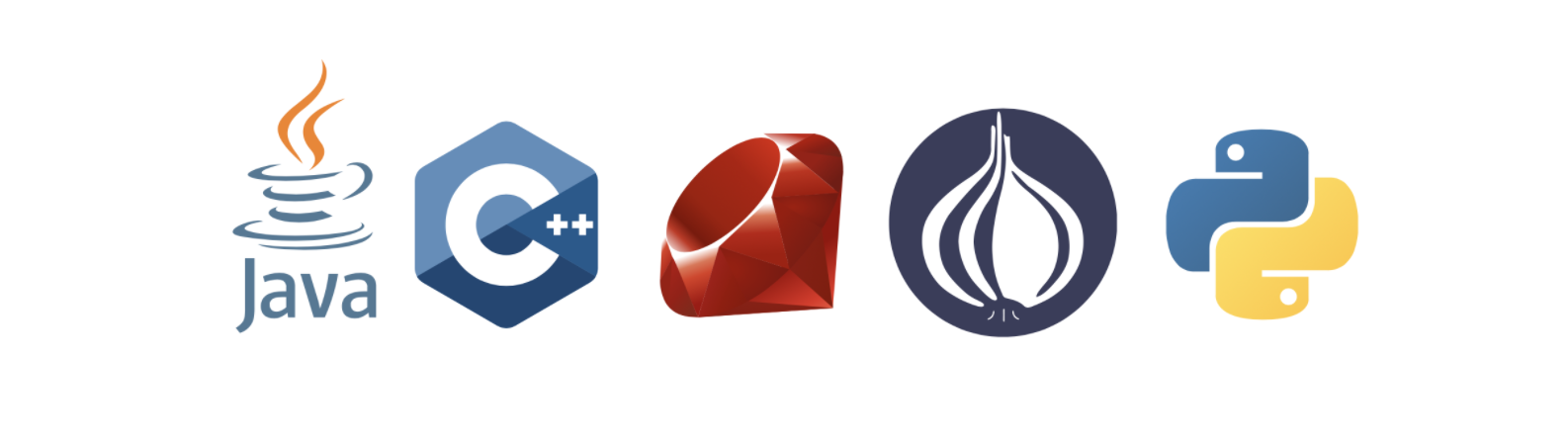• At Facebook, you can expect these questions no matter what level you are interviewing for.

Structured interviewing:

• Facebook will pair you with interviewers who have either held the position you’re interviewing for or with individuals who work directly with the position you’re interviewing for.

Core values and your behavioral interview:

• Facebook interviewers will also evaluate your ability to embody their five core values: Move Fast, Be Bold, Focus on Impact, Be Open, and Build Social Value.

## Top 40 Facebook coding interview questions

In this section, we’ll take a deep dive into the top 40 coding interview questions. We will discuss the answers and runtime complexities for the 15 questions you’re bound to see in an interview followed by the definitive list of 25 questions you’ll likely encounter.

As you can see to the right, Facebook focuses mostly on arrays and strings. These are essential skills to master.

Each question will be solved in Python 3. To see these solutions in C++, Ruby, Java, and JavaScript, visit here.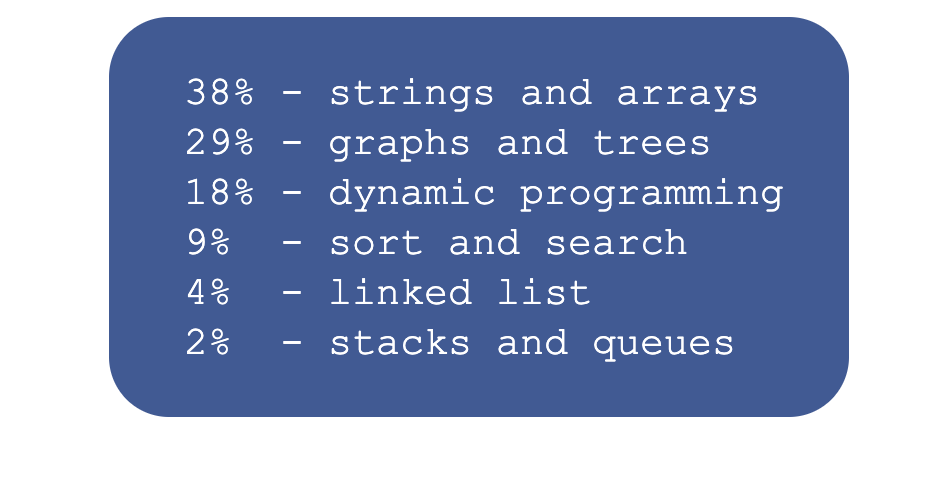### Arrays: move zeros to the left

Given an integer array, move all elements that are 0 to the left while maintaining the order of other elements in the array. The array has to be modified in-place. Try it yourself before reviewing the solution and explanation.1. Take this integer array
1 of 2
def move_zeros_to_left(A):  #TODO: Write - Your - Code  pass

Runtime complexity: Linear, $O(n)$

Memory Complexity: Constant, $O(1)$

Keep two markers: read_index and write_index and point them to the end of the array. Let’s take a look at an overview of the algorithm.

While moving read_index towards the start of the array:

• If read_index points to 0, skip.
• If read_index points to a non-zero value, write the value at read_index to write_index and decrement write_index.
• Assign zeros to all the values before the write_index and to the current position of write_index as well.

### Arrays: Merge overlapping intervals

You are given an array (list) of interval pairs as input where each interval has a start and end timestamp. The input array is sorted by starting timestamps. You are required to merge overlapping intervals and return a new output array.

Consider the input array below. Intervals (1, 5), (3, 7), (4, 6), (6, 8) are overlapping so they should be merged to one big interval (1, 8). Similarly, intervals (10, 12) and (12, 15) are also overlapping and should be merged to (10, 15).

Try it yourself before reviewing the solution and explanation.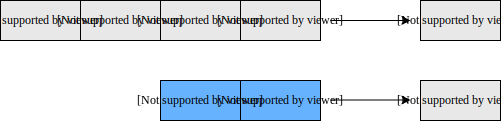class Pair:  def __init__(self, first, second):    self.first = first    self.second = seconddef merge_intervals(v):  # write your code here  result = []  return result

Runtime complexity: Linear, $O(n)$

Memory Complexity: Linear, $O(n)$

This problem can be solved in a simple linear scan algorithm. We know that input is sorted by starting timestamps. Here is the approach we are following:

• List of input intervals is given, and we’ll keep merged intervals in the output list.
• For each interval in the input list:
• If the input interval is overlapping with the last interval in the output list then we’ll merge these two intervals and update the last interval of the output list with the merged interval.
• Otherwise, we’ll add an input interval to the output list.

### Trees: Convert binary tree to doubly linked list

Convert a binary tree to a doubly linked list so that the order of the doubly linked list is the same as an in-order traversal of the binary tree.

After conversion, the left pointer of the node should be pointing to the previous node in the doubly linked list, and the right pointer should be pointing to the next node in the doubly linked list. Try it yourself before reviewing the solution and explanation.1. Consider this tree
1 of 2
main.py
binary_tree.py
binary_tree_node.py
from binary_tree import *from binary_tree_node import *def convert_to_linked_list(root):    # TODO: Write - Your - Code    return root

Runtime complexity: Linear, $O(n)$

Memory Complexity: Linear, $O(h)$.

Recursive solution has $O(h)$ memory complexity as it will consume memory on the stack up to the height of binary tree h. It will be $O(log n)$ for balanced trees and in the worst case can be $O(n)$.

In an in-order traversal, first the left sub-tree is traversed, then the root is visited, and finally the right sub-tree is traversed.

One simple way of solving this problem is to start with an empty doubly linked list. While doing the in-order traversal of the binary tree, keep inserting each element output into the doubly linked list.

But, if we look at the question carefully, the interviewer wants us to convert the binary tree to a doubly linked list in-place i.e. we should not create new nodes for the doubly linked list.

This problem can be solved recursively using a divide and conquer approach. Below is the algorithm specified.

• Start with the root node and solve left and right subtrees recursively
• At each step, once left and right subtrees have been processed:
• Fuse output of left subtree with root to make the intermediate result
• Fuse intermediate result (built in the previous step) with output from the right subtree to make the final result of the current recursive call.

### Trees: Level order traversal of binary tree

Given the root of a binary tree, display the node values at each level. Node values for all levels should be displayed on separate lines. Let’s take a look at the below binary tree.

Level order traversal for this tree should look like:

• 100
• 50, 200
• 25, 75, 350

main.py
binary_tree.py
binary_tree_node.py
# Import required functionsfrom collections import dequefrom binary_tree import *from binary_tree_node import *def level_order_traversal(root):    result = ""    # TODO: Write - Your - Code    print(result, end="")

Runtime complexity: Linear, $O(n)$

Memory Complexity: Linear, $O(n)$

Here, you are using two queues: current_queue and next_queue. You push the nodes in both queues alternately based on the current level number. You’ll dequeue nodes from the current_queue, print the node’s data, and enqueue the node’s children to the next_queue.

Once the current_queue becomes empty, you have processed all nodes for the current level_number. To indicate the new level, print a line break (\n), swap the two queues, and continue with the above-mentioned logic.

After printing the leaf nodes from the current_queue, swap current_queue and next_queue. Since the current_queue would be empty, you can terminate the loop.

### Strings: Reverse words in a sentence

Reverse the order of words in a given sentence (an array of characters). Take the “Hello World” string for example: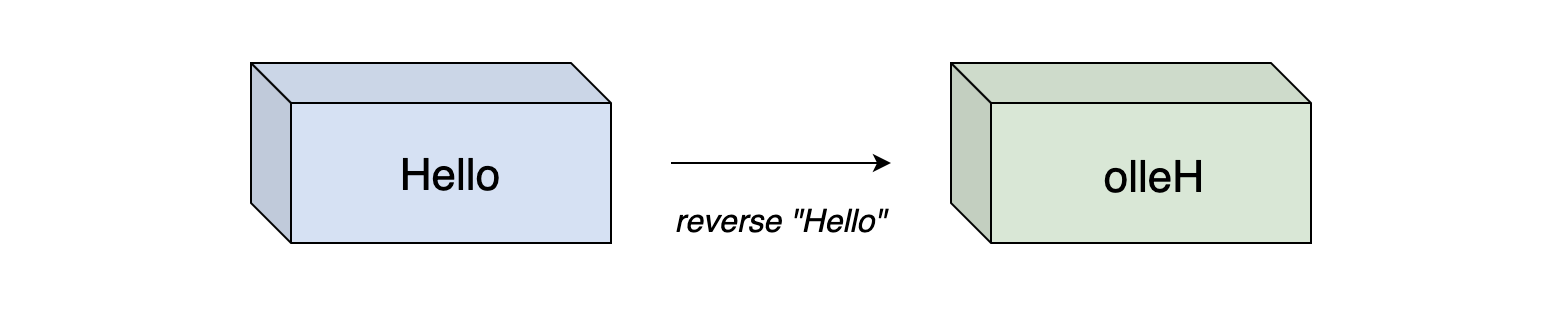def reverse_words(sentence):    # sentence here is an array of characters  #TODO: Write - Your - Code  return sentence

Here is how the solution works:

• Reverse the string.
• Traverse the string and reverse each word in place.

For more on string reversal, read my article Best practices for reversing a string in JavaScript, C++, and Python.

### Strings: String segmentation

You are given a dictionary of words and a large input string. You have to find out whether the input string can be completely segmented into the words of a given dictionary. The following example elaborates on the problem further.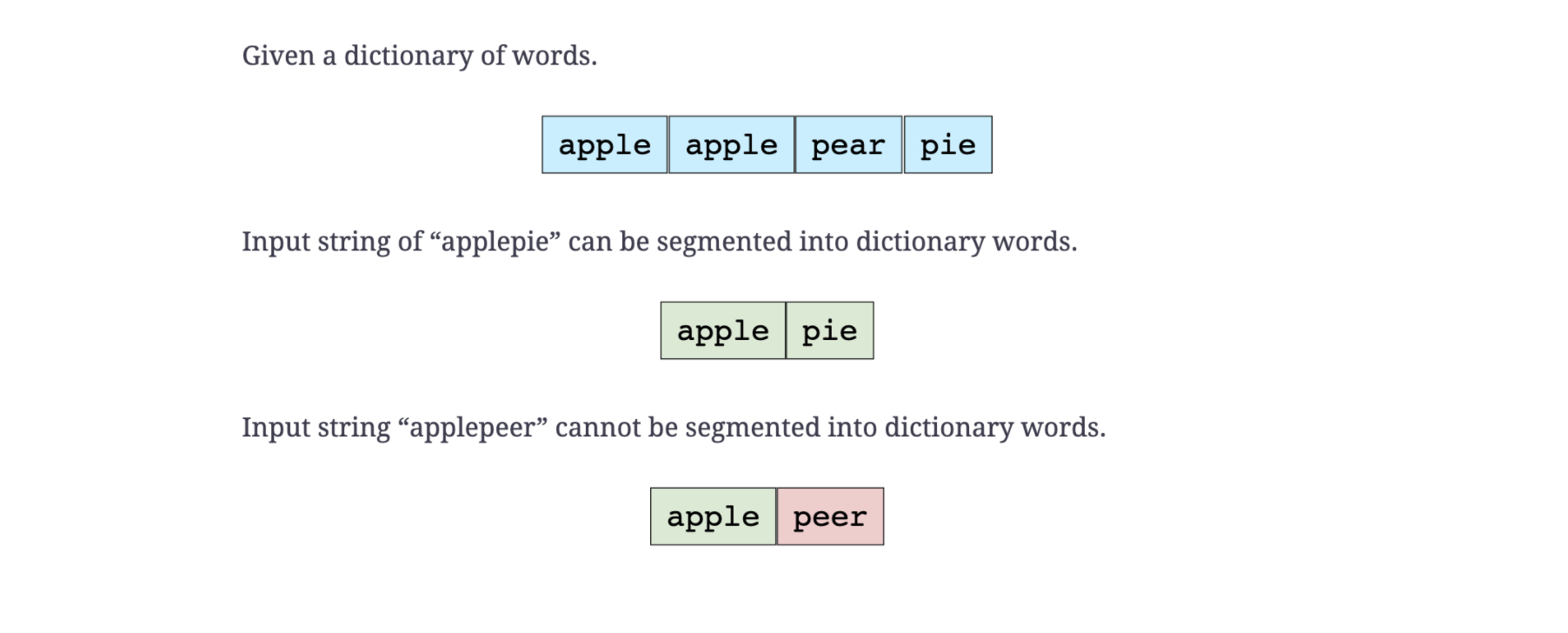def can_segment_string(s, dictionary):  #TODO: Write - Your - Code  return False

Runtime complexity: Exponential, $O(2^{n})$, if we only use recursion. With memoization, the runtime complexity of this solution can be improved to be polynomial, $O(n^{2})$.

Memory Complexity: Polynomial, $O(n^{2})$

You can solve this problem by segmenting the large string at each possible position to see if the string can be completely segmented to words in the dictionary. If you write the algorithm in steps it will be as follows:

n = length of input string
for i = 0 to n - 1
first_word = substring (input string from index [0, i] )
second_word = substring (input string from index [i + 1, n - 1] )
if dictionary has first_word
if second_word is in dictionary OR second_word is of zero length, then return true
recursively call this method with second_word as input and return true if it can be segmented


The algorithm will compute two strings from scratch in each iteration of the loop. Worst case scenario, there would be a recursive call of the second_word each time. This shoots the time complexity up to $2^{n}$.

You can see that you may be computing the same substring multiple times, even if it doesn’t exist in the dictionary. This redundancy can be fixed by memoization, where you remember which substrings have already been solved.

To achieve memoization, you can store the second string in a new set each time. This will reduce both time and memory complexities.

### Dynamic Programming: Find maximum single sell profit

Given a list of daily stock prices (integers for simplicity), return the buy and sell prices for making the maximum profit.

We need to maximize the single buy/sell profit. If we can’t make any profit, we’ll try to minimize the loss. For the below examples, buy (orange) and sell (green) prices for making a maximum profit are highlighted.

def find_buy_sell_stock_prices(array):  #TODO: Write - Your - Code  return -1, -1 #Return a tuple with (high, low) price values

Runtime complexity: Linear, $O(n)$

Memory Complexity: Constant, $O(1)$

The values in the array represent the cost of a stock each day. As we can buy and sell the stock only once, we need to find the best buy and sell prices for which our profit is maximized (or loss is minimized) over a given span of time.

A naive solution, with runtime complexity of $O(n^{2})$, is to find the maximum gain between each element and its succeeding elements.

There is a tricky linear solution to this problem that requires maintaining current_buy_price (which is the smallest number seen so far), current_profit, and global_profit as we iterate through the entire array of stock prices.

At each iteration, we will compare the current_profit with the global_profit and update the global_profit accordingly.

The basic algorithm is as follows:

current buy = stock_prices
global sell = stock_prices
global profit = global sell - current buy

for i = 1 to stock_prices.length:
current profit = stock_prices[i] - current buy
if current profit is greater than global profit
then update global profit to current profit and update global sell to stock_prices[i]
if stock_prices[i] is less than current buy
then update current buy to stock_prices[i]

return global profit and global sell


### Math and Stats: Calculate the power of a number

Given a double, x, and an integer, n, write a function to calculate x raised to the power n. For example:

power (2, 5) = 32

power (3, 4) = 81

power (1.5, 3) = 3.375

power (2, -2) = 0.25

def power(x, n): #TODO: Write - Your - Code  return x

Runtime complexity: Logarithmic, $O(log n)$

Memory Complexity: Logarithmic, $O(log n)$

A simple algorithm for this problem is to multiply x by n times. The time complexity of this algorithm would be $O(n)$. We can use the divide and conquer approach to solve this problem more efficiently.

In the dividing step, we keep dividing n by 2 recursively until we reach the base case i.e. n == 1

In the combining step, we get the result, r, of the sub-problem and compute the result of the current problem using the two rules below:

• If $n$ is even, the result is $r * r$ (where $r$ is the result of sub-problem)
• If $n$ is odd, the result is $x * r * r$ (where $r$ is the result of sub-problem).

### Backtracking: Find all possible subsets

We are given a set of integers and we have to find all the possible subsets of this set of integers.

def get_all_subsets(v, sets):  #TODO: Write - Your - Code  return sets

Runtime complexity: Exponential, $O(2^{n} * n)$, where $n$ is the number of integers in the given set

Memory Complexity: Constant, $O(2^{n} * n)$

There are several ways to solve this problem. We will discuss the one that is neat and easier to understand. We know that for a set of ‘n’ elements there are $2^{n}$​​ subsets. For example, a set with 3 elements will have 8 subsets. Here is the algorithm we will use:

n = size of given integer set
subsets_count = 2^n
for i = 0 to subsets_count
form a subset using the value of 'i' as following:
bits in number 'i' represent index of elements to choose from original set,
if a specific bit is 1 choose that number from original set and add it to current subset,
e.g. if i = 6 i.e 110 in binary means that 1st and 2nd elements in original array need to be picked.
add current subset to list of all subsets


Note that the ordering of bits for picking integers from the set does not matter; picking integers from left to right would produce the same output as picking integers from right to left.

### Graphs: Clone a directed graph

Given the root node of a directed graph, clone this graph by creating its deep copy so that the cloned graph has the same vertices and edges as the original graph.

Let’s look at the below graphs as an example. If the input graph is G = (V, E) where V is set of vertices and E is set of edges, then the output graph (cloned graph) G’ = (V’, E’) such that V = V’ and E = E’.

Note: We are assuming that all vertices are reachable from the root vertex. i.e. we have a connected graph.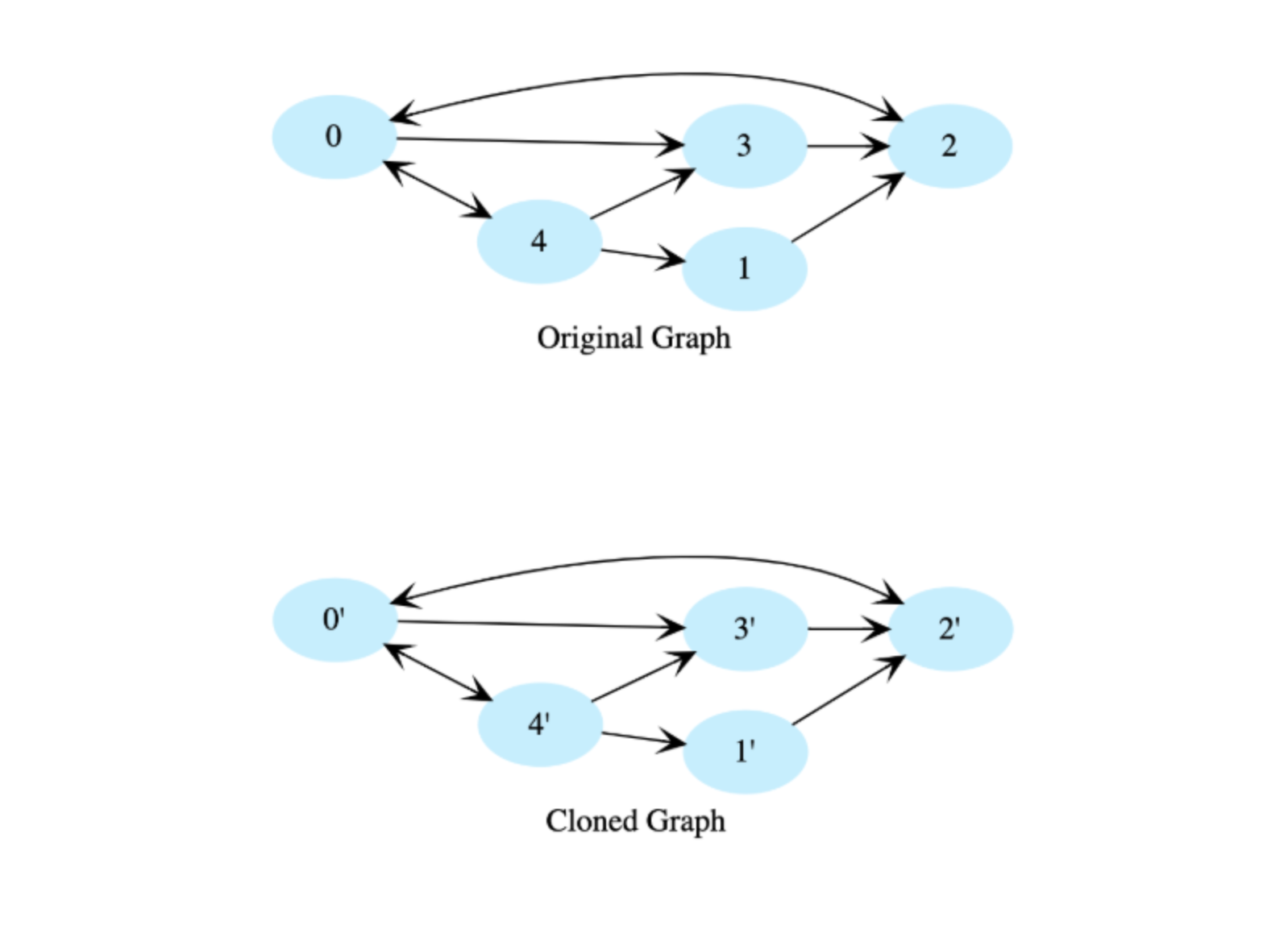main.py
directed_graph.py
node.py
from directed_graph import *def clone(graph):  # Hashmap to keep record of visited nodes  nodes_completed = {}  # Creating new graph  clone_graph = DirectedGraph()  # TODO: Write - Your - Code    # Return deep copied graph  return clone_graph

Runtime complexity: Linear $O(n)$

Memory Complexity: Logarithmic, $O(n)$

We use depth-first traversal and create a copy of each node while traversing the graph. To avoid getting stuck in cycles, we’ll use a hashtable to store each completed node and will not revisit nodes that exist in the hashtable.

The hashtable key will be a node in the original graph, and its value will be the corresponding node in the cloned graph.

### Design: Serialize / deserialize binary tree

Serialize a binary tree to a file and then deserialize it back to a tree so that the original and the deserialized trees are identical.

• Serialize: write the tree in a file.
• Deserialize: read from a file and reconstruct the tree in memory.

There is no restriction regarding the format of a serialized stream, therefore you can serialize it in any efficient format. However, after deserializing the tree from the stream, it should be exactly like the original tree. Consider the below tree as the input tree.

main.py
binary_tree.py
binary_tree_node.py
from binary_tree import *from binary_tree_node import *# Function to serialize tree into list of integer.def serialize(root):  #TODO: Write - Your - Code  return None# Function to deserialize integer list into a binary tree.def deserialize(stream):   #TODO: Write - Your - Code  return None

Runtime complexity: Linear $O(n)$

Memory Complexity: Logarithmic, $O(logn)$

There can be multiple approaches to serialize and deserialize the tree. One approach is to perform a depth-first traversal and serialize individual nodes to the stream. We’ll use a pre-order traversal here. We’ll also serialize some markers to represent a null pointer to help deserialize the tree.

Consider the below binary tree as an example. Markers (M*) have been added in this tree to represent null nodes. The number with each marker i.e. 1 in M1, 2 in M2, merely represents the relative position of a marker in the stream.

The serialized tree (pre-order traversal) from the above example would look like the below list.

When deserializing the tree we’ll again use the pre-order traversal and create a new node for every non-marker node. Encountering a marker indicates that it was a null node.

### Sorting and Searching: Find the high and low index

Given a sorted array of integers, return the low and high index of the given key. You must return -1 if the indexes are not found.

The array length can be in the millions with many duplicates.

In the following example, according to the the key, the low and high indices would be:

• key: 1, low = 0 and high = 0
• key: 2, low = 1 and high = 1
• key: 5, low = 2 and high = 9
• key: 20, low = 10 and high = 10

For the testing of your code, the input array will be:

1, 1, 1, 2, 2, 2, 2, 2, 3, 3, 3, 4, 4, 4, 4, 5, 5, 5, 6, 6, 6, 6, 6, 6

def find_low_index(arr, key): #TODO: Write - Your - Code  return -2def find_high_index(arr, key):  #TODO: Write - Your - Code  return -2

Runtime complexity: Logarithmic $O(log n)$

Memory Complexity: Constant, $O(1)$

Linearly scanning the sorted array for low and high indices are highly inefficient since our array size can be in millions. Instead, we will use a slightly modified binary search to find the low and high indices of a given key. We need to do binary search twice:

• Once for finding the low index.
• Once for finding the high index.

#### Low index

Let’s look at the algorithm for finding the low index:

• At every step, consider the array between low and high indices and calculate the mid index.
• If the element at mid index is less than the key, low becomes mid + 1 (to move towards the start of range).
• If the element at mid is greater or equal to the key, the high becomes mid - 1. Index at low remains the same.
• When low is greater than high, low would be pointing to the first occurrence of the key.
• If the element at low does not match the key, return -1.

#### High index

Similarly, we can find the high index by slightly modifying the above condition:

• Switch the low index to mid + 1 when the element at mid index is less than or equal to the key.
• Switch the high index to mid - 1 when the element at mid is greater than the key.

### Sorting and Searching: Search rotated array

Search for a given number in a sorted array, with unique elements, that has been rotated by some arbitrary number. Return -1 if the number does not exist.

Assume that the array does not contain duplicates

Below is an original array before rotation.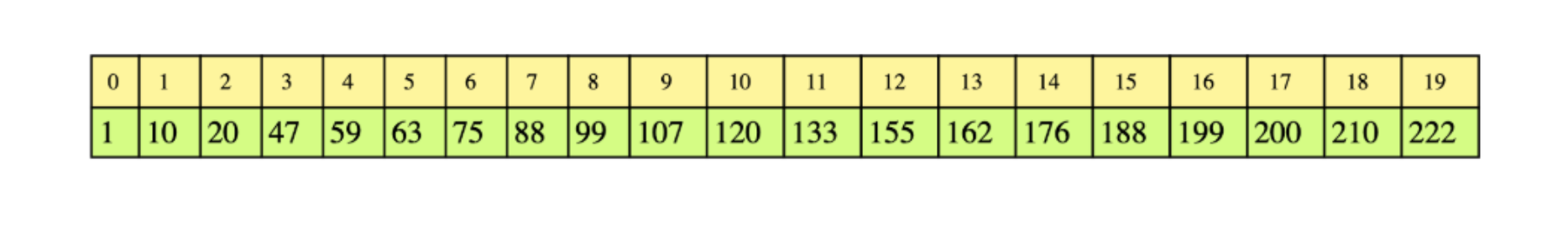After performing rotation on this array 6 times it changes to: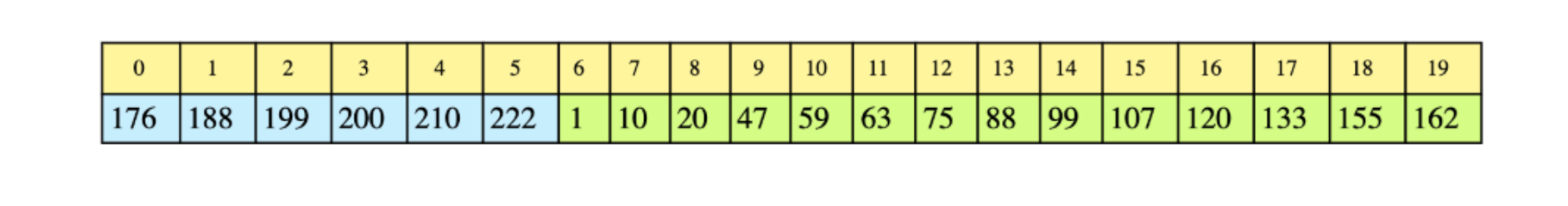The task is to find a given number in this array.

def binary_search_rotated(arr, key):  #TODO: Write - Your - Code  return -1

Runtime complexity: Logarithmic, $O(log n)$

Memory Complexity: Logarithmic, $O(log n)$

The solution is essentially a binary search but with some modifications. If we look at the array in the example closely, we notice that at least one half of the array is always sorted. We can use this property to our advantage.

If the number n lies within the sorted half of the array, then our problem is a basic binary search. Otherwise, discard the sorted half and keep examining the unsorted half. Since we are partitioning the array in half at each step, this gives us $O(log n)$ runtime complexity.

### 25 more common Facebook coding interview questions

1. Longest increasing subsequence from array of integers (dynamic programming arrays)
2. Unique paths in a grid (dynamic programming matrices)
3. Add two numbers as a list (lists)
4. Design cache (system design)
5. Design a highly consistent database (system design)
6. Rotate a matrix (arrays)
7. Design a URL shortener (system design)
8. Design a recommendation system (ML, system design)
9. Find nth Fibonacci number (number theory)
10. Find the square root of an integer using binary search (math search answer)
11. Implement StrStr (string search)
12. Minimum appends for Palindrome (strings)
13. Find the largest rectangle in a histogram (stacks)
1. Substring concatenation (incremental hash)
2. Find the least common ancestor (tree search)
3. Find largest distance between nodes in a tree (DFS)
4. Find all unique triplets in an array, giving sum of zero (array)
5. Find maximum path sum in non-empty binary tree (binary tree)
6. Find K closest points to origin for a list of points on a plane (search/sort)
7. Write a function to compute intersection of arrays (sort/search)
8. Design a typehead feature (system design)
9. Design Facebook Messenger (system design)
10. Group anagrams together in an array of strings (arrays/strings)
11. Convert a BST to sorted circular doubly linked list (trees)
12. Determine the order of letters in a dictionary (graphs/trees)## How to prepare for a Facebook interview

Now that you have a sense of what to expect from an interview and know what kinds of questions to expect, let’s learn some preparation strategies based on Facebook’s unique interview process.

The first thing you should do is update your resume to be metrics/deliverables driven. It’s also a good idea to show how the work you’ve done can translate into their five core values: Move fast, Be bold, Focus on impact, Be open, and Build social value.

### Practice generalist coding questions

I recommend at least three months of self-study to be successful. This includes choosing a programming language, reviewing the basics, and studying algorithms, data structures, system design, object-oriented programming, and more.

It’s important to practice coding using different tools:

• Simple Text Editor (like CoderPad)
• By hand (on a whiteboard or paper)
• Your preferred style of coding

For a robust, 12-week interview guide, check out our article, the 3 Month Coding Interview Preparation Bootcamp

The design interview usually doesn’t involve any coding, so you’ll need to learn how to answer these questions. This will be done on a whiteboard during the interview, so practice your designs by hand. Study up on system design and product design.

The best way to master system design questions is not by memorizing answers but by learning the anatomy of a system design question. You need to train yourself to think from the ground up while also considering scaling and requirements.

Pro tip: If you want to stand out in the system design interview, you’ll need to discuss how Machine Learning can be implemented in your design.

Facebook wants next-gen engineers, and they focus heavily on artificial intelligence. Consider brushing up on ML concepts and ML system design principles.

### Master the best practices

Once you get the basics down and progress through the interview prep roadmap, master the best practices.

When you practice, learn how to articulate your process out loud. Facebook cares a lot about how you think. When you code, explain your thought process as if another person were in the room.

You also want to start timing yourself to learn how to manage your time effectively. It’s always better to take time planning your answer than to just jump it with brute force.

### Prepare for behavioral interviews

Facebook cares that you fit with their company, so you need to be prepared to talk about yourself. For each of Facebook’s values, brainstorm how you fit and why these values matter to you.

You should also think about your 2 to 4 year career aspirations, interests, and strengths as an engineer, as they will likely come up in the interview.

To learn how to prepare, check out my article Behavioral Interviews: how to prepare and ace interview questions

### Prepare questions for your interviewers

Facebook values self-starters, so it’s important that you come prepared with questions for your interviewers. You’ll have time during every interview to ask your own questions. This is also an opportunity to determine if Facebook is a good fit for your lifestyle and needs.

## Wrap up and resource list

Cracking the Facebook coding interview comes down to the time you spend preparing, such as practicing coding questions, studying behavioral interviews, and understanding Facebook’s company culture.

There is no golden ticket, but more preparation will surely make you a more confident and desirable candidate. The essential resources below will help you prepare and build confidence for Facebook interviews.

Keep learning and studying!

### Learn the patterns behind common interview questions